Home > INT3 > Chapter 11 > Lesson 11.1.2 > Problem11-19

11-19.
1. Multiply or divide the expressions below. Simplify your results. Homework Help ✎

1.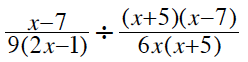2.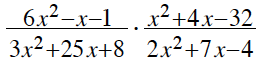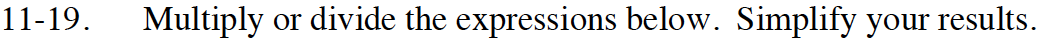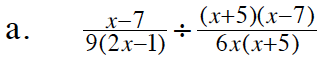Take the reciprocal of (flip) the divisor (the fraction on the right side) and look for ones to factor out.

$\frac{2x}{3(2x-1)}\text{ or } \frac{2x}{6x-3}$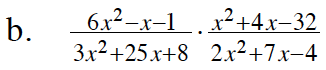Factor each of the trinomials. (They all factor.) Look for ones to factor out. Multiply the factors that remain.

$\frac{x-4}{x+4}$